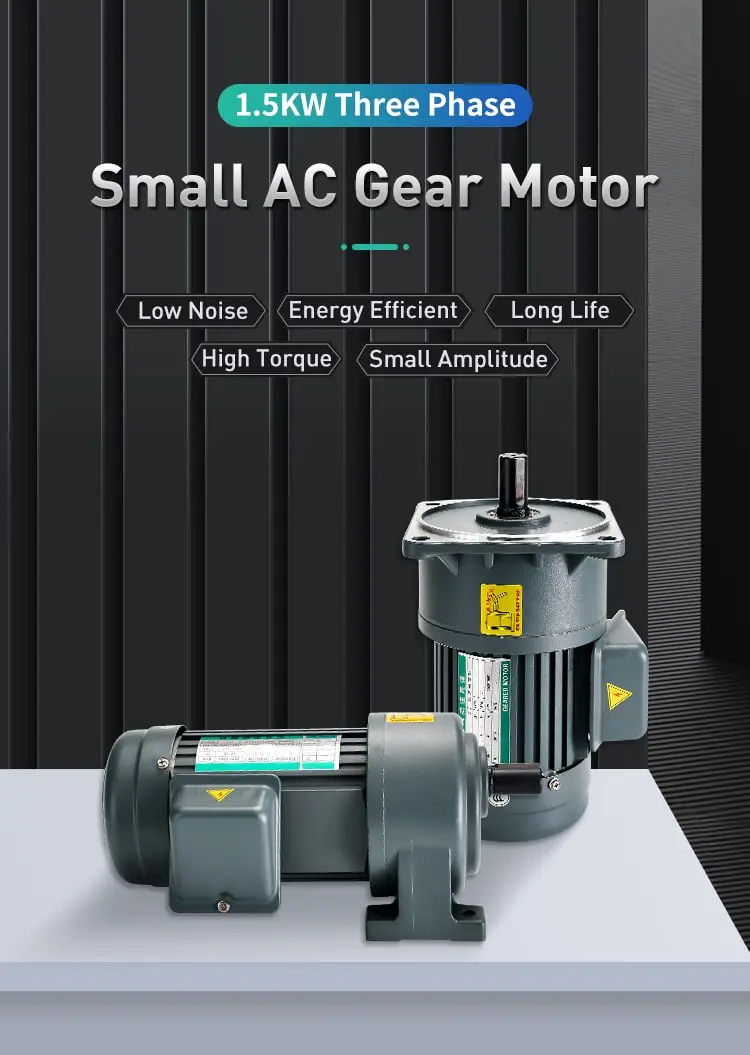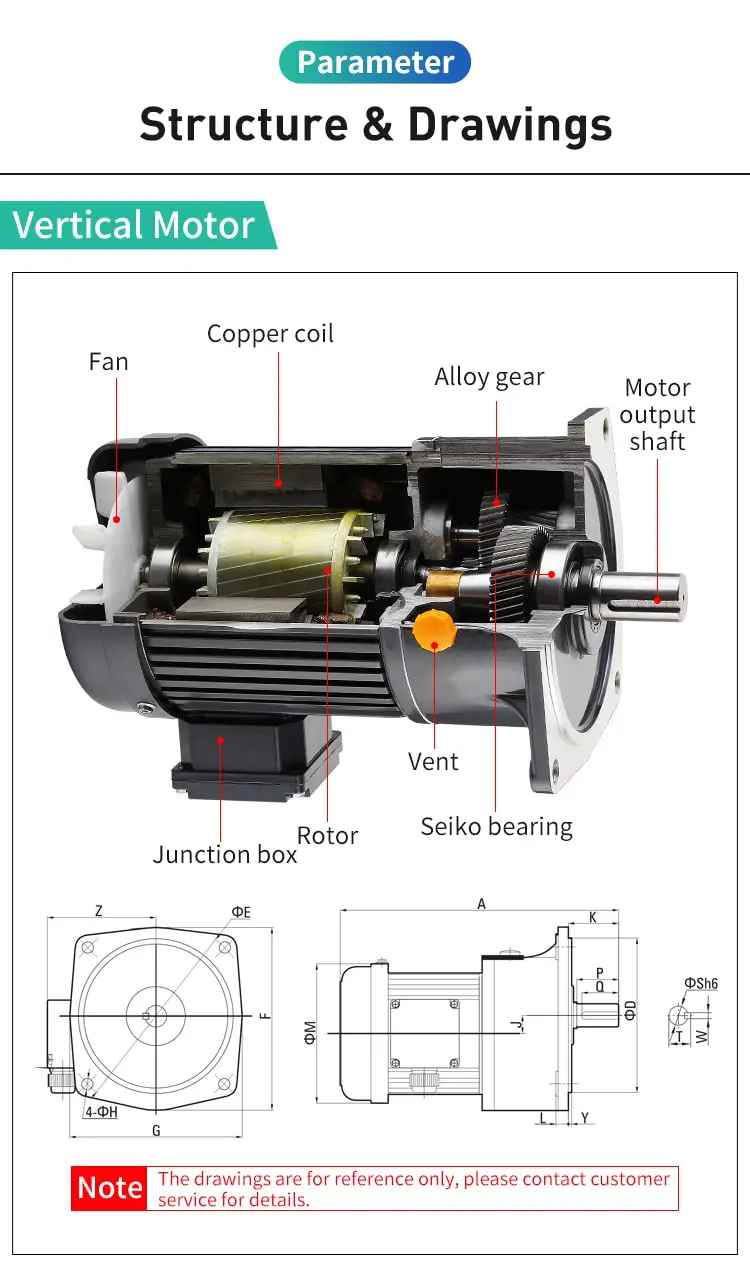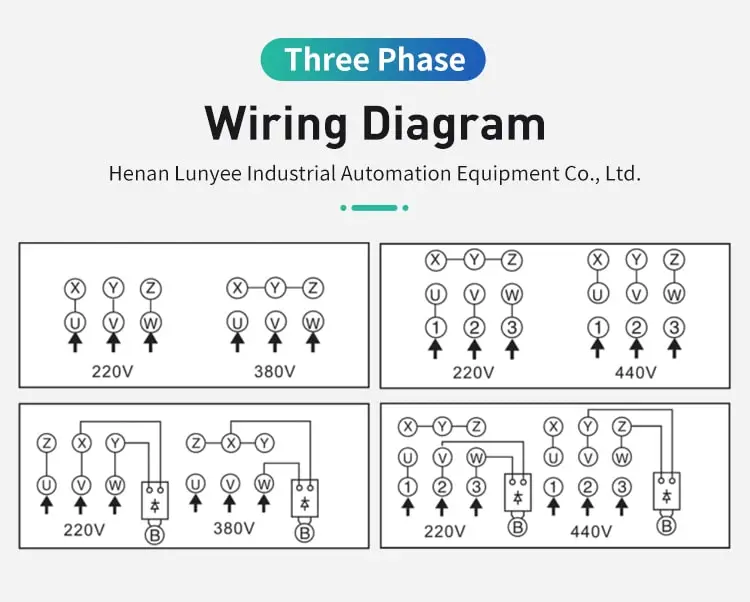By Jasmine 2023-03-27 10:28:15

#### How to find synchronous speed of induction motor?

Determining the synchronous speed of an induction motor is an important step in evaluating its performance and efficiency. Synchronous speed can be accurately calculated by following the appropriate steps for single-phase or three-phase motors.

Synchronous speed is a crucial parameter of an induction motor, which is defined as the speed at which the rotating magnetic field produced by the stator winding of the motor rotates. It is a function of the frequency and number of poles of the motor, and it is the speed at which the rotor would rotate if it were perfectly synchronized with the rotating magnetic field. In this essay, we will explore how to find the synchronous speed of an induction motor.

The formula to calculate synchronous speed is:

Synchronous Speed = 120 x Frequency / Number of Poles

Where frequency is the supply frequency of the motor and the number of poles is the number of magnetic poles present in the stator winding.

To find the synchronous speed of an induction motor , we first need to determine the frequency and the number of poles of the motor. The frequency is typically the same as the supply voltage frequency, which is either 50 Hz or 60 Hz, depending on the region. The number of poles is determined by the design of the stator winding and is typically a multiple of 2.

Once the frequency and number of poles are known, we can calculate the synchronous speed using the formula mentioned above. For example, consider a 3-phase induction motor with a supply frequency of 50 Hz and a stator winding with 4 poles. Using the formula, we get:

Synchronous Speed = 120 x 50 / 4 = 1500 RPM

This means that the rotating magnetic field produced by the stator winding of this motor rotates at a speed of 1500 RPM. If the rotor of the motor were perfectly synchronized with the rotating magnetic field, it would also rotate at 1500 RPM. However, due to slip, the rotor rotates at a slightly lower speed than the synchronous speed.

It is important to note that the synchronous speed of an induction motor is only achievable when the motor is running with no load and is not affected by any external factors such as load torque or changes in the supply voltage or frequency. In practice, the actual speed of the motor is always slightly lower than the synchronous speed due to various losses in the motor and the effect of load torque.

In conclusion, finding the synchronous speed of an induction motor is essential for understanding its performance characteristics and designing the motor for a specific application. The synchronous speed can be calculated using the formula based on the frequency and number of poles of the motor. It is important to keep in mind that the actual speed of the motor is always slightly lower than the synchronous speed due to slip and other losses.